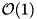STXXL  1.4.1
STXXL Deque

The acronym Deque stands for "double-ended-queue", that means elements can be accessed, inserted and deleted on both ends of the data structure - in contrast to the stxxl::queue (see STXXL Queue), where that's only possible on one of the two endings.

### Creating a STXXL deque

To create a stxxl::deque object, only the data value type must be specified:

typedef stxxl::deque<int> deque;
deque my_deque;

See stxxl::deque for additional template parameter details.

### Insert elements

Inserting elements is possible at the start (by calling the push_front() function) as well as the end (by calling the push_back() function) of the deque.

my_deque.push_front(2);
my_deque.push_front(11);
my_deque.push_back(5);
my_deque.push_back(8);
// deque now stores: |11|2|5|8|

### Access elements

To return a reference to the element at the start of the deque, call front(), to return a reference to the elemtent at the end of the deque, call back() on a deque instance.

std::cout << "return 'first' element: " << my_deque.front() << std::endl; // prints 11
std::cout << "return 'last' element: " << my_deque.back() << std::endl; // prints 8

Accessing elements at random is supported by the STXXL deque with the []-operator like the following.

std::cout << "random access: " << my_deque << std::endl; // prints 5

The operations described in this sections are not I/O-efficient as they come withtime per I/O-access. To achieve I/O-efficient scanning, the STXXL deque provides different iterators. The simplest iterator can be used as follows:

// create forward-iterator (which advances from start to end)
stxxl::deque_iterator<deque> deque_iterator = my_deque.begin();
// access item at current iterator position
std::cout << *deque_iterator << std::endl;
// move up one step by preincrement
++deque_iterator;

### Delete elements

Deleting elements is possible at both endings of the deque by using pop_front() and pop_back():

my_deque.pop_front(); // deque now stores: |2|5|8|
my_deque.pop_back(); // deque now stores: |2|5|

### Determine size / Check whether the deque is empty

To determine the size (i.e. the number of elements) of an instance, call size():

std::cout << "deque stores: " << my_deque.size() << " elements" << std::endl;

To check if the deque is empty, call empty() which returns true if the deque is empty:

std::cout << "empty deque? " << my_deque.empty() << std::endl;

### A minimal example on STXXL's deque

(See examples/containers/deque1.cpp for the sourcecode of the following example).

#include <stxxl/deque>
#include <iostream>
int main()
{
typedef stxxl::deque<int> deque;
deque my_deque;
my_deque.push_front(2);
my_deque.push_front(11);
my_deque.push_back(5);
my_deque.push_back(8);
// deque now stores: |11|2|5|8|
std::cout << "return 'first' element: " << my_deque.front() << std::endl; // prints 11
std::cout << "return 'last' element: " << my_deque.back() << std::endl; // prints 8
std::cout << "random access: " << my_deque << std::endl; // prints 5
// generate forward iterator
stxxl::deque_iterator<deque> deque_iterator = my_deque.begin();
// iterate over my_deque, access values and delete them afterwards
while (!my_deque.empty())
{
std::cout << *deque_iterator << " ";
++deque_iterator;
my_deque.pop_front();
}
return 0;
}

See examples/containers/deque2.cpp for the sourcecode of a more comprehensive example.